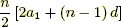# Week 10 In Precalculus 11

This week in Precalculus was a review in preparation for our midterm. Three thing that I needed to review was solving radical expressions, converting standard to general form and our first chapter “Sequences and Series”.Above I have shown two questions one showing how to find the sum of a series, and one finding the the last term using the sum given in the question, by using the formulato find the sum and  an = a1 + (n – 1)d to find the nth term.